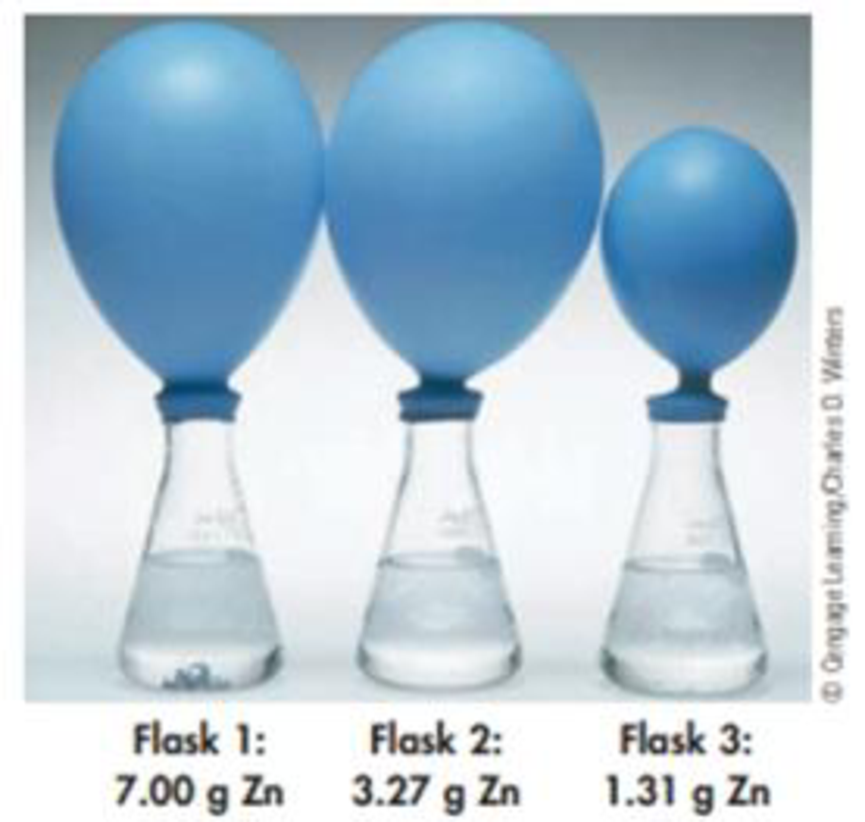# Let us explore a reaction with a limiting reactant. Here, zinc metal is added to a flask containing aqueous HCl, and H 2 gas is a product. Zn(s) + 2 HCl(aq) → ZnCl 2 (aq) + H 2 (g) The three flasks each contain 0.100 mol of HCl. Zinc is added to each flask in the following quantities. When the reactants are combined, the H 2 inflates the balloon attached to the flask. The results are as follows: Flask 1: Balloon inflates completely, but some Zn remains when inflation ceases. Flask 2: Balloon inflates completely. No Zn remains. Flask 3: Balloon does not inflate completely. No Zn remains. Explain these results. Perform calculations that support your explanation.### Chemistry & Chemical Reactivity

9th Edition
John C. Kotz + 3 others
Publisher: Cengage Learning
ISBN: 9781133949640

#### Solutions

Chapter
Section### Chemistry & Chemical Reactivity

9th Edition
John C. Kotz + 3 others
Publisher: Cengage Learning
ISBN: 9781133949640
Chapter 4, Problem 137SCQ
Textbook Problem
168 views

## Let us explore a reaction with a limiting reactant. Here, zinc metal is added to a flask containing aqueous HCl, and H2 gas is a product.Zn(s) + 2 HCl(aq) → ZnCl2(aq) + H2(g)The three flasks each contain 0.100 mol of HCl. Zinc is added to each flask in the following quantities.When the reactants are combined, the H2 inflates the balloon attached to the flask. The results are as follows:Flask 1: Balloon inflates completely, but some Zn remains when inflation ceases.Flask 2: Balloon inflates completely. No Zn remains.Flask 3: Balloon does not inflate completely. No Zn remains.Explain these results. Perform calculations that support your explanation.

Interpretation Introduction

Interpretation:

The reason for the difference in inflammation of balloons on the basis of limiting reagent involved in the reaction has to be explained.

Concept introduction:

• For chemical reaction balanced chemical reaction equation written in accordance with the Law of conservation of mass.
• Law of conservation of mass states that for a reaction total mass of the reactant and product must be equal.
• Numberofmole=GivenmassofthesubstanceMolarmass
• The molar mass of an element or compound is the mass in grams of 1 mole of that substance, and it is expressed in the unit of grams per mol (g/mol).
• Stoichiometric factor is a relationship between reactant and product which is obtained from the balanced chemical equation for a particular reaction.
• Limiting reagent: limiting reagent is a reactant, which consumes completely in the chemical reaction. The quantity of the product depends on this limiting reagent, it can be determined with the help of balanced chemical equation for the reaction.

### Explanation of Solution

Inflammation of balloon is mainly due to the hydrogen production inside the flask.

When zinc reacts with hydrogen chloride, zinc chloride and hydrogen will be produced.

Balanced equation for the reaction is,

Zn(s)+2HCl(aq)ZnCl2(aq)+H2(g)

The balanced chemical equation indicates that the stoichiometric ratio of HCl to Zn is 2molHCl:1molZn. In each reaction there is 0.100molHCl present.

In flask 1: Balloon inflates completely, but some Zn remains when inflammation ceases.

The reaction in flask 1, there is 0.107molZn present, and it is calculated by using the equation,

Numberofmole=GivenmassofthesubstanceMolarmass

Amount of Zn =  7.0065

### Still sussing out bartleby?

Check out a sample textbook solution.

See a sample solution

#### The Solution to Your Study Problems

Bartleby provides explanations to thousands of textbook problems written by our experts, many with advanced degrees!

Get Started

Find more solutions based on key concepts
B vitamins often act as: a. antioxidants. b. blood clotting factors. c. coenzymes. d. none of the above.

Nutrition: Concepts and Controversies - Standalone book (MindTap Course List)

Speed is a(n) ___ quantity. (2.2)

An Introduction to Physical Science

Why do biologists often use model organisms in their research?

Biology: The Dynamic Science (MindTap Course List)

Write the structural formula for ethyl propyl ether.

Introductory Chemistry: An Active Learning Approach

Can more water vapor be held in warm air or cool air?

Oceanography: An Invitation To Marine Science, Loose-leaf Versin

The position of a pinewood derby car was observed at various times; the results are summarized in the following...

Physics for Scientists and Engineers, Technology Update (No access codes included)